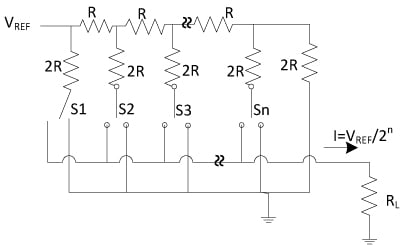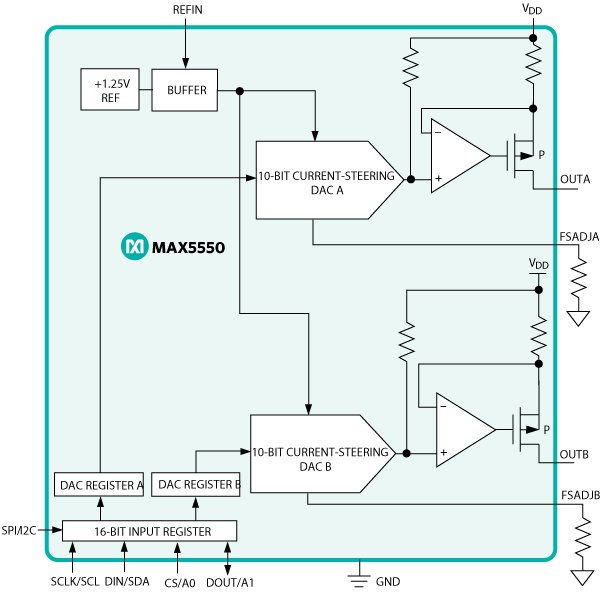# Applications of Current DACs

### 要約

This application note discusses the typical architecture of current DACs and their specific applications. It also discusses how to select them for adjusting/margining power-supply voltage, biasing PIN diode, and controlling tunable laser transmitter as well as the gain and attenuation of Semiconductor Optical Amplifier (SOA)

### Introduction

The worldwide use of the electronic devices creates high demands for DACs to connect digital systems to the analog world such as the fiber optical communication networks, to bias photo diodes or to digitally control analog devices such as power supplies to precisely deliver stable, high-resolution currents from the very low microamps to hundreds of milliamps. The output stage of a DAC can be designed to provide a voltage or current output. This application note discusses the current output type and its intended applications.

### Current DAC Architecture

#### What is a DAC?

A digital-to-analog converter (also known as a DAC or a D/A converter) is an electronic device that converts a digital representation of quantity into a discrete analog value. The input to the DAC is typically a digital binary code. This code, along with a known reference voltage, results in a voltage or current at the DAC's output. The word "discrete" is very important to understand because a DAC cannot provide a continuous-time output signal. Instead of that, it provides analog "steps". The steps can be low pass filtered to obtain a continuous signal. By increasing the resolution of the DAC, the number of discrete steps is increased, and the step size is reduced (which reduces the quantization error). This operation results in a signal that closely approximates a continuous-time signal.

Figure 1 shows the typical current DAC architecture. This current DAC comprises a series of R-2R resistors and switches. As the interface digital input code is commanded, the internal 2R leg resistors are correspondingly directed to VREF or ground via the switches. The output following through the load is current of which the value is proportional to VREF/2n. Where n is the number of selected switches.#### DS4402 Current DAC

Figure 2 shows the functional diagram of the DS4402/DS4404 current DAC.

The DS4402/DS4404 are two and four I2C adjustable current DACs, respectively. Each device can sink or source the current. Each output has 31 sinks and 31 source settings, which are controlled by the I2C interface, and the external resistors RFS0 to RFS3 set the full-scale range and step size of each output.#### Applications of DS4402/DS4404

The DS4402/DS4404 can sink and source the current up to ±2mA, making them ideal current DACs for applications such as power-supply adjustment, power-supply margining, and adjustable current sink or source.

Figure 3 shows the schematic of the DS4402/DS4404 used to control the power supply output voltage with ±20% margin. The adjustable power supply has a DC-DC converter output voltage, VOUT, of 2.0V and a DC-DC converter feedback voltage, VFB, of 0.8V.

The values of R0A and R0B resistors are determined as follows:

VFB = VOUT × R / (R0A + R0B)

Where, VOUT = 2.0V and VFB = 0.8V

Substitute VOUT and VFB from equation 2 to equation 1 to obtain equation 3.

0.8 = 2.0 × R0B / (R0A + R0B)

Rearrange the equation 3 to obtain equation 4.

R0A = 1.5 × R0B

Choose IOUT0 to be 0.8mA, which is approximately the midrange source and sink current of the DS4402/DS4404.

From Figure 3, IOUT0 = I0A – I0B

Also, from Figure 3, I0B = VFB / R0B

I0A = (VOUT – VFB) / R0A

Substitute equations 4, 6 and 7 to equation 5 to obtain the following equation 8.

R0B = [(VOUT – VFB) / 1.5 – VFB] / IOUT0

To achieve a 20% margin in the power supply voltage, set VOUT to 2.4V.

R0B is calculated to be 333O, and R0A is calculated to be 500O, based on the equation 8. In this configuration, with ±0.8mA of sourcing and sinking current capability, the DS4402/DS4404 current DACs allow the output voltage to be moved linearly from 1.6V to 2.4V.#### MAX5550 Current DAC

The MAX5550 dual, 10-bit DAC features high-output-current up to 30mA per DAC, which makes it ideal for applications in PIN diode biasing that require approximately 10mA to 20mA DC current.

Figure 4 shows the MAX5550 functional diagram.The radio frequency (RF) attenuation is commonly required in wireless designs as a variable attenuator to reduce or increase the RF signal to an appropriate level to meet the system requirements. Figure 5 shows a typical PIN diode biasing circuit. When the DC bias current from the MAX550 increases, the forward resistance of a PIN diode decreases, which reduces the attenuation in the RF signal path. Similarly, when the DC bias current from the MAX550 decreases, the forward resistance of a PIN diode increases, which in turn increases the attenuation in the RF signal path.

A system controller adjusts the PIN diode resistance (RPIN) value by varying the current sourcing from the MAX5550 through the PIN diode. Therefore, the signal attenuation can be controlled by using the MAX5550 current DAC.#### MAX5112/MAX5113 Current DAC

Tunable laser diodes require high precision current source with high current output, low noise, and low digital feedthrough. The MAX5112/MAX5113 buffered current DACs are specifically designed and particularly suited for tunable laser control as they can drive a tunable laser up to 300mA and provide other attractive features that tunable laser diodes require. The MAX5112 and MAX5113 are designed to interface with I2C and SPI, respectively.

Figure 6 shows the 14-bit MAX5112/MAX5113 DACs feature 9-channel, current-output DACs. The devices operate from a low +3.0V power supply and provide 14-bit performance without any adjustment. The output ranges of the devices are optimized to bias a high-power tunable laser source. Each of the 9 channels provides a current source ranging from 2mA to 300mA. In addition, the MAX5112/MAX5113 are designed to provide additional current or to achieve higher resolution by connecting the DAC outputs in parallel.

The MAX5112/MAX5113 also offer low noise density at full scale of only 0.5nA/vHz at 2mA and 56nA/vHz at 300mA and digital interface feedthrough is kept low of approximately 4LSB as shown in Figure 7 to ensure low jitter operation for laser diode applications.#### Applications of MAX5112/MAX5113 Current DACs

The MAX5112/MAX5113 is designed to provide both current source up to 300mA and current sink as low as -60mA. This attractive feature allows to control a semiconductor optical amplifier (SOA) which amplifies incident light through stimulated emission to either set the gain by sourcing current up to 300mA to compensate for the transmission loss or set the attenuation by sinking current of up to -60mA as shown in Figure 8.Figure 9 shows the Lumentum LambdaFLEX Tunable 10G TOSA. This high-performance tunable laser transmitter incorporates a 14-bit, multichannel current output DAC with an I2C interface to the laser, SOA, and M-Z functions.### Conclusion

Current DACs are available in different output current levels, i.e., low, medium, and high. The low current DAC can provide output in µA to a couple of mA, the medium device can provide output in the order of tens of mA, and the high-output current part can supply output in hundreds of mA. They are specifically designed for different applications. The low-current output DACs as the DS4402/DS4404 are made to adjust the power-supply output voltage optimization. The medium-current output DAC such as the MAX5550 is designed for PIN diode biasing which requires only up to 20mA of current. The high-current output DAC such as the MAX5112/MAX5113 which are capable of sourcing and sinking current up to 300mA and as low as -60mA are specifically created for high-performance tunable laser transmitter and controlling the gain and attenuation of SOA.

### References

1. Lumentum LambdaFLEX Tunable 10G TOSA